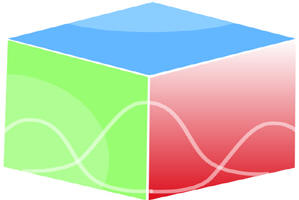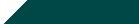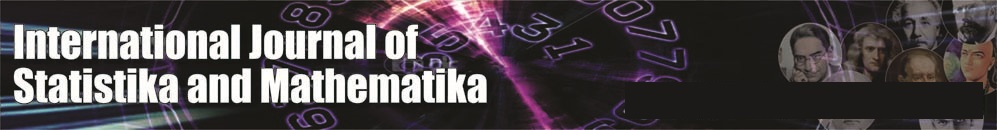``Home| Journals | Statistics Online Expert | About Us | Contact UsUntitled Document

[

On Vanishing of Pure Extensions of Torsion-Free Locally Compact Abelian Groups

H. Sahleh1, A. A. Alijani2

1,2 Department of Mathematics, Faculty of Mathematical Sciences, University of Guilan, P.O. BOX 1914.Rasht, IRAN.

Research Article

Abstract: Let  be the class of all torsion-free, locally compact abelian (LCA) groups. In this paper, we determine the LCA groups  such that every pure extension  splits for each  in .

keywords: Pure extension, split, torsion-free,locally compact abelian group.

MSC 2000: Primary 22B05, Secondary   22A05

# 1.Introduction

Throughout, all groups are Hausdorff abelian topological groups and will be written additively. Let  £ denote the category of locally compact abelian (LCA) groups with continuous homomorphisms as morphisms. The Pontrjagin dual of a group  is denoted by .  A morphism is called proper if it is open onto its image, and a short exact sequence  in  £ is said to be proper exact if  and are proper morphisms. In this case, the sequence is called an extension of  by . A subgroup of a group  is called pure if  for all positive integers . An extension  is called a pure extension if  is pure in . Following Fulp and Griffith , we let  denote the (discrete) group of extensions of  by . A subgroup of a group is called pure if  for all positive integers . The elements represented by pure extensions of  by  form a subgroup of  which is deenoted by . Assume that  is any subcategory of  £. This paper is part of an investigation which answers the following question:

under what conditions on , or  for all Some answers are given in , in the category of locally compact abelian groups. Let  be the category of all torsion-free, locally compact abelian groups. In , Fulp has determined the LCA groups  such that  for all  . In this paper, we determine the discrete or compact groups  satisfying  for all .

The additive topological group of real numbers is denoted by  ,  is the group of rationals ,  is the group of integers and  is the cyclic group of order . By  we mean the group  with discrete topology and   is the torsion part of . The topological isomorphism will be denoted by . For more on locally compact abelian groups, see .

# 2.Splitting in the category of torsion-free, locally compact abelian groups

In this section, we determine the structure of a discrete or a compact group  such that  for all torsion-free groups £.

Recall that a discrete group A is said to be cotorsion if for any discrete torsion-free group B , Ext(B,A)=0.

Lemma 2.1  for all torsion-free groups £.

Proof. Let  be a discrete torsion-free group. By [1, Theorem 52.3],  where  is a divisible hull of . By [1, Theorem 46.1 and Theorem 54.6], and so is a reduced,algeraically compact and cotorsion group. It follows from [1, Prposition 54.2] that

Now suppose that  is a torsion-free group in  £.

Then .□

Theorem 2.2  Let  be a discrete group. Then  for all torsion-free groups £  if and only if  where  is a discrete torsion group.

Proof. Let  be a torsion-free group. By [2, Proposition 4], we have the following exact sequence: Since  is torsion and a torsion-free group, so  Now assume that . By (1), . It follows from [3, Proposition 2] that . By [1, Theorem 28.2], .

Conversely, suppose that  where

is discrete torsion . If  is torsion-free, then

It is enough to show that . There

exists a pure exact sequence

where  is a direct sum of finite cyclic groups and, , a divisible torsion groupS. Consider the exact sequence

Since , so by lemma 2.1, . Clearly

. So .□

Lemma 2.3 Let  and  be two topological spaces,  and an open map. If , then  is open.

Proof. It is clear.□

Theorem 2.4 Let  be a pure, closed and torsion-free subgroup of . Then  for all .

Proof.  Let  be the natural map. For a fixed , we show that  is a topological isomorphism. Assume that . Then . Since  is pure,  for some . So  for some . Therefore,  and ,that is  is surjective. Now suppose that  for some . Then  and . Since  is torsion-free, . So  is injective. Since  is open and  is injective, so by lemma 2.3,  is open. Hence .□

Definition 2.5 Let be an extension in  £. Then  is said to be pure if the sequence

is  proper exact for all . Following Loth , we let  denote the group of pure extensions of  by .

Lemma 2.6 Let £. Then

for all torsion-free groups £.

Proof. Let  be a pure extension of torsion-free group  by . We may assume that  is the inclusion homomorphism. So  is a pure, closed and torsion-free subgroup of . Now by Theorem 2.4,  for all . Since so  is proper exact for all .

Theorem 2.7 Let  be a compact group. Then  for all torsion-free groups if and only if  is torsion.

Proof. Assume that  is a compact group such that  for each torsion-free group . By [7, Lemma 2.13], . So . Since the sequence

Is exact, . Now consider the exact sequence

So  is isomorphic to  and therefore . It follows that  is torsion. Since  , so  . Hence  is cotorsion. By [1, Corollary 54.4],  where  is a bounded group and  a divisible group. So . Clearly,  for each torsion-free group . Consequently by [3, Theorem 2],  or .

Conversely, let  be a compact torsion group. Then by [6, Theorem 4.2] and Lemma 2.6,  for all torsion-free groups .

# References

1. L. Fuchs,  Infinite abelian groups, Vol. I, Academic Press, New York, 1970.
2. R.O. Fulp,  Homological study of purity in locally compact groups, Proc.London Math. Soc.21, pp 501-512(1970).
3. R.O. Fulp,  Splitting locally compact abelian groups, Michigan Math. J.19, pp 47-55(1972).
4. E. Hewitt and K. Ross, Abstract Harmonic Analysis, Vol I, Second Edition, Springer Verlag, Berlin, 1979.
5. R.O Fulp and P. Griffith, Extensions of locally compact abelian groups I, Trans. Amer. Math.Soc.154,pp 341-356(1971).
6. P. Loth, Topologically pure extensions abelian groups, Rings and modules, Proceedings of the AGRAM 2000 Conference in Perth, Western Australia, July 9-15,2000, Contemporary Mathematics 273, American Mathematical Society, pp 191-201(2001).
7. P. Loth, Pure extensions of locally compact abelian groups, Rend. Sem. Mat. Univ. Padova. 116, pp 31-40 (2006).